# reactor desigen Q3 For the following reaction 2A + A+B is reported as follows: T, deg....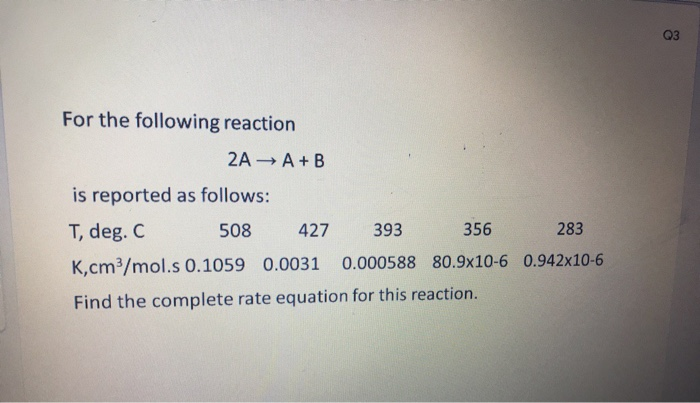reactor desigen
Q3 For the following reaction 2A + A+B is reported as follows: T, deg. C 508 427 393 356 283 Kcm/mol.s 0.1059 0.0031 0.000588 80.9x10-6 0.942x10-6 Find the complete rate equation for this reaction.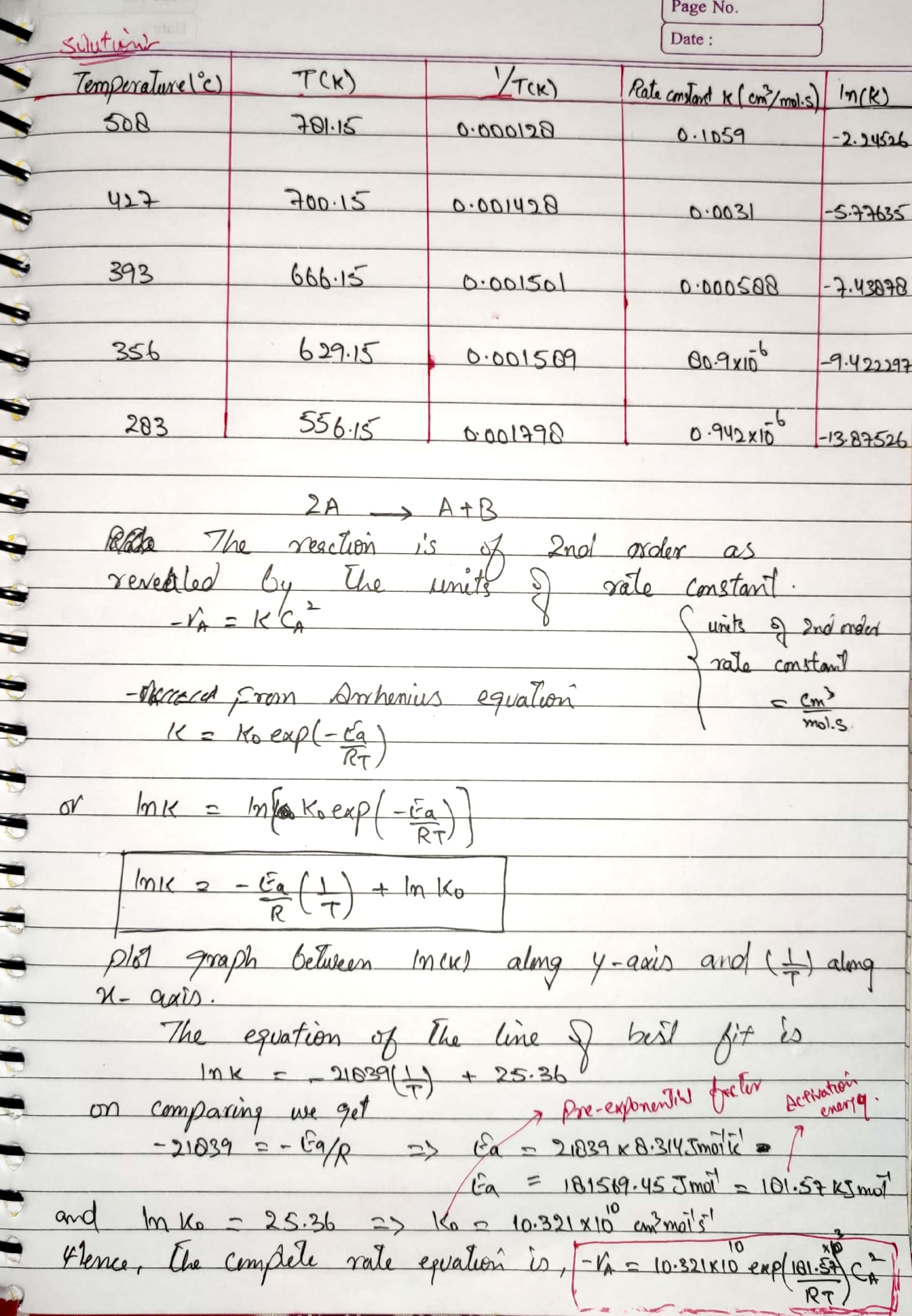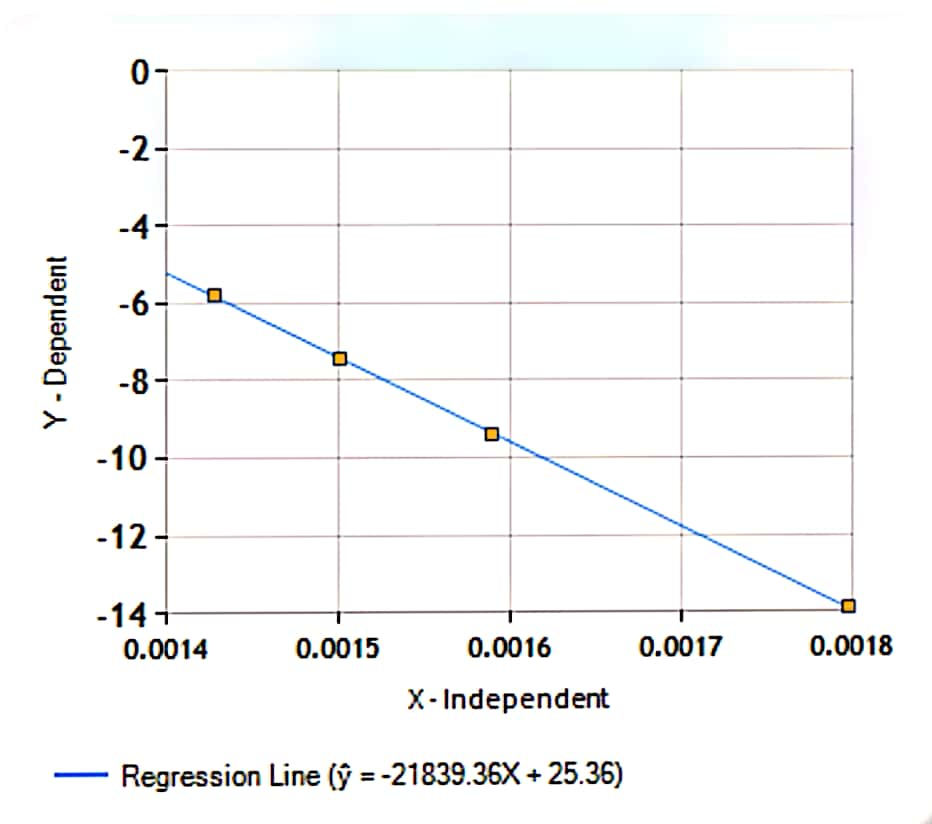##### Add Answer of: reactor desigen Q3 For the following reaction 2A + A+B is reported as follows: T, deg....
Similar Homework Help Questions
• ### For Biochemical engineering - Interpretation of batch reactor.

3.31 The thermal decomposition of hydrogen iodide,2HI -> H2 + I2is reported by M .Bodenstein as follows;T (*C)508427393356283k (cm3/mol.s)0.10590.003100.00058880.9x10-6 0.942x10-6Find the complete rat equation for this reaction. Use unit of joules, moles, cm3 and seconds.

• ### For the reaction 2A --> B + ½ C, answer the following questions: a.If –rA =...

For the reaction 2A --> B + ½ C, answer the following questions: a.If –rA = 10 mol L-1 min-1, calculate the rate of reaction with respect to Band C, rB and rC, respectively. b.10 moles of A are fed to a reactor. If 70% conversion occurs, calculate the moles of all chemical species. Are the total moles conserved during the course of reaction? c.The reaction order with respect to A is 2.0. Provide the units that would be possessed...

• ### this is matlab.. updated, this is not matlab. 2. Saponification batch reactor. In a saponification reaction that t...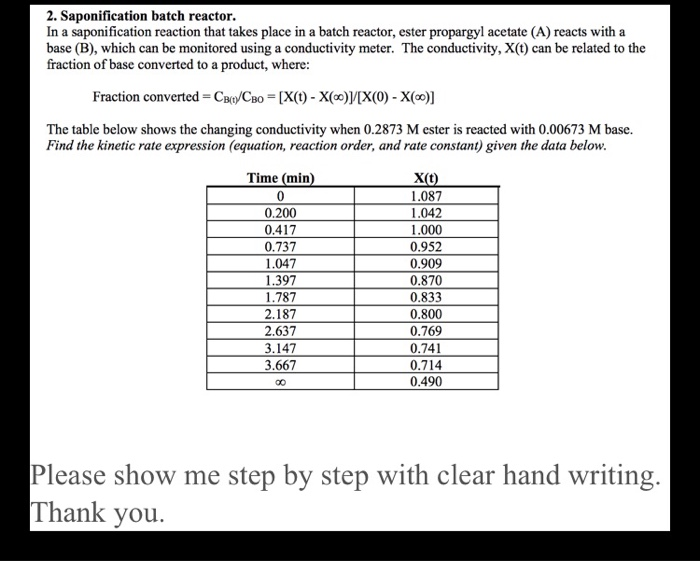this is matlab.. updated, this is not matlab. 2. Saponification batch reactor. In a saponification reaction that takes place in a batch reactor, ester propargyl acetate (A) reacts with a base (B), which can be monitored using a conductivity meter. The conductivity, X(t) can be related to the fraction of base converted to a product, where: Fraction converted = CBt/CBo = [X(t) - X(0) [X(0) - X(0)] The table below shows the changing conductivity when 0.2873 M ester is reacted...

• ### change with temperature. 10. (10 points, due Th 31 Oct) For the reaction 2A + B...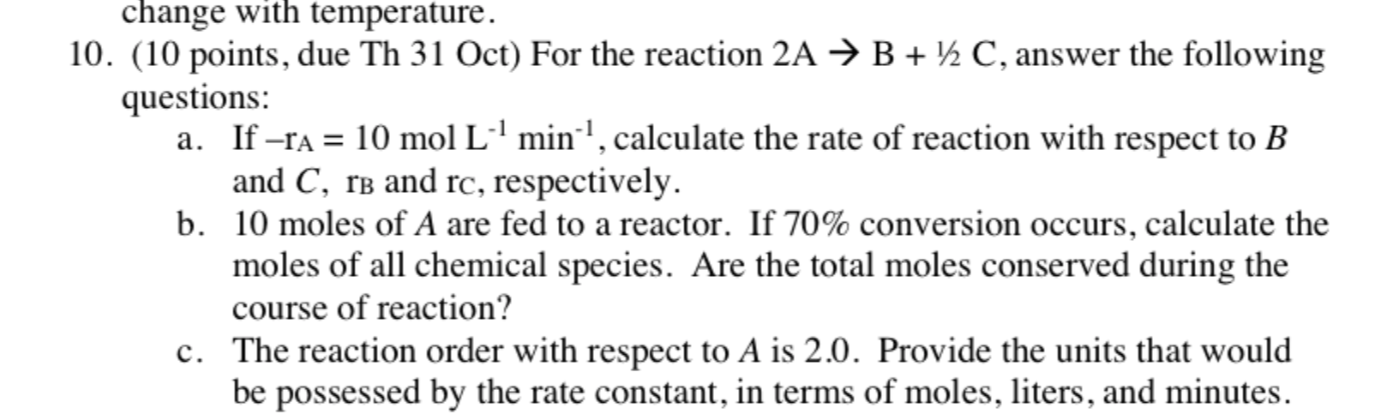change with temperature. 10. (10 points, due Th 31 Oct) For the reaction 2A + B + 12 C, answer the following questions: a. If –ra = 10 mol Lº'min', calculate the rate of reaction with respect to B and C, rb and rc, respectively. b. 10 moles of A are fed to a reactor. If 70% conversion occurs, calculate the moles of all chemical species. Are the total moles conserved during the course of reaction? c. The reaction order...

• ### For the following reaction: 2A + 3B --> 2C relate the rate of reaction for all three substances

For the following reaction: 2A + 3B --> 2C relate the rate of reaction for all three substancesChoose one answer.a. 2delta[A]/delta(t) = 3delta[B]/delta(t) = -2delta[C]/delta(t)b. -1/2delta[A]/delta(t) = -1/3delta[B]/delta(t) = 2delta[C]/delta(t)c. -2delta[A]/delta(t) = -3delta[B]/delta(t) = 2delta[C]/delta(t)d. 1/2delta[A]/delta(t) = 1/3delta[B]/delta(t) = -1/2delta[C]/delta(t)After performing several experiments you determine that the rate law for the reaction 2A + 3B --> 2C is:Rate = k[A]2[B]This rate law indicates that the reactions is:Choose one answer.a. First order for reactant A.b. First order for reactant B.c. Second...

• ### The homogeneous gas reaction 2A ---> 3R follows second-order kinetics. For a feed rate of 4m3/...

The homogeneous gas reaction 2A ---> 3R follows second-order kinetics. For a feed rate of 4m3/hr of pure A at 5 atm and 350oC, an experimental reactor consisting of a 2.5 cm ID pipe 2 m long gives 65% conversion of feed. A commercial plant is to treat 310 m3/hr of feed consisting of 50% A, 50% inerts at 25 atm and 350 degrees C to obtain 80% conversion. Assume plug flow in the pipe, negligible pressure drop, and ideal...

• ### 5. The following reaction is a second order irreversible reaction: 2A + 3B2C i. Derive an...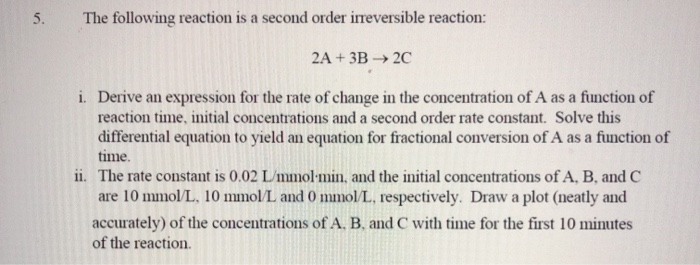5. The following reaction is a second order irreversible reaction: 2A + 3B2C i. Derive an expression for the rate of change in the concentration of A as a function of reaction time, initial concentrations and a second order rate constant. Solve this differential equation to yield an equation for fractional conversion of A as a function of time The rate constant is 0.02 L mmol min, and the initial concentrations of A, B, and C are 10 mmol/L, 10...

• ### 9,10,11 9. Consider the decomposition reaction of N2Os. 2 N203(g) → 4NO2(g) + O2(g) follows the...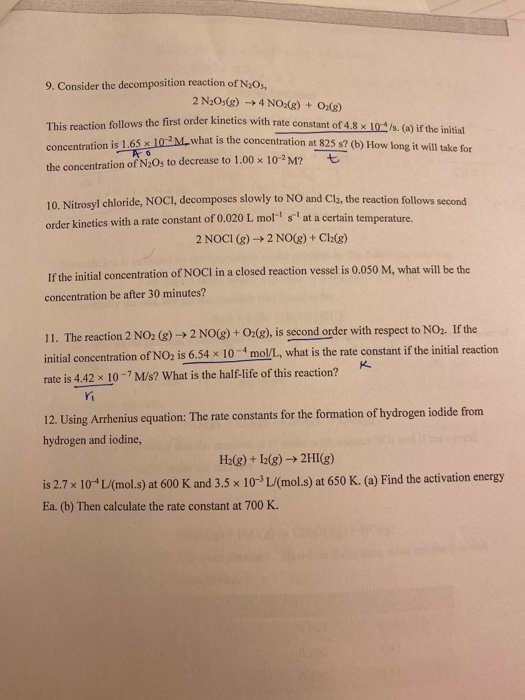9,10,11 9. Consider the decomposition reaction of N2Os. 2 N203(g) → 4NO2(g) + O2(g) follows the first order kinetics with rate constant of 4.8 x 10's. (a) if the initial 165 x 10-2M what is the concentration at 825 s? (b) How long it will take for concentration is 1.65 x 10-2M what is the concentrat the concentration of N2Os to decrease to 1.00 x 10-2M? t 10. Nitrosyl chloride, NOCI, decomposes slowly to NO and Cl2, the reaction follows...

• ### The rate law for the reaction 2A + B - C is found to be rate...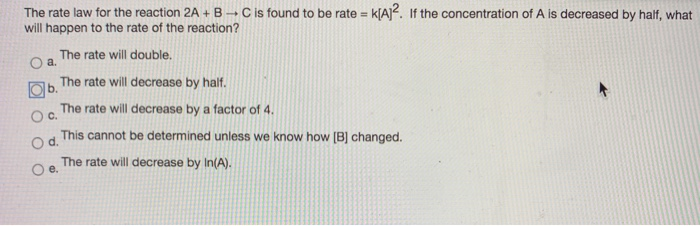The rate law for the reaction 2A + B - C is found to be rate = k[A]2. If the concentration of A is decreased by half, what will happen to the rate of the reaction? O a. The rate will double. The rate will decrease by half. The rate will decrease by a factor of 4. O d. This cannot be determined unless we know how [B] changed. The rate will decrease by In(A). b. C. e. a. Which...

• ### Consider the following mechanism. step 1 2A > B slow B+ C D fast step 2 overall: 2A+C D Determine the rate law for t...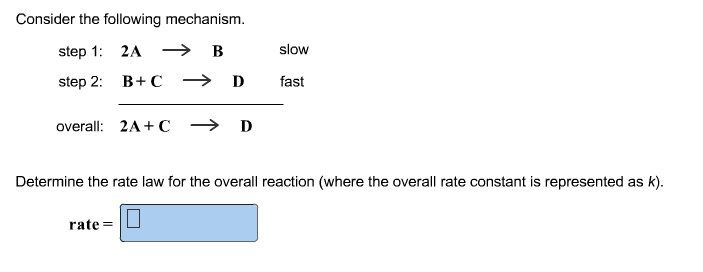Consider the following mechanism. step 1 2A > B slow B+ C D fast step 2 overall: 2A+C D Determine the rate law for the overall reaction (where the overall rate constant is represented as k) rate=

Free Homework App